# 1568 A Challenging Christmas Tree

### Today’s Puzzle:

Can you write the numbers from 1 to 10 in each of the boldly outlined columns and rows so that each quadrant of this Christmas tree puzzle behaves like a multiplication table? Remember to use logic and not guessing and checking.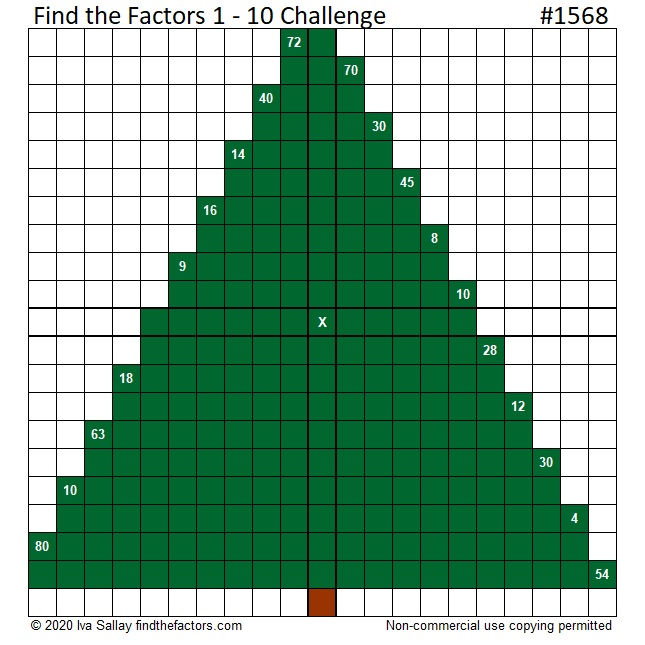Here is the same puzzle that won’t use so much ink to print:### 1568 Factor Tree:

Here is one of several possible factor trees for 1568: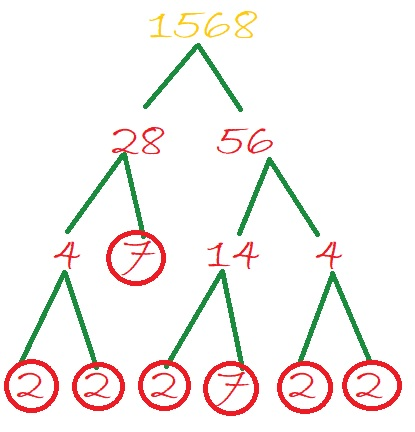### Factors of 1568:

• 1568 is a composite number.
• Prime factorization: 1568 = 2 × 2 × 2 × 2 × 2 × 7 × 7, which can be written 1568 = 2⁵ × 7².
• 1568 has at least one exponent greater than 1 in its prime factorization so √1568 can be simplified. Taking the factor pair from the factor pair table below with the largest square number factor, we get √1568 = (√784)(√2) = 28√2.
• The exponents in the prime factorization are 5 and 2. Adding one to each exponent and multiplying we get (5 + 1)(2 + 1) = 6 × 3 = 18. Therefore 1568 has exactly 18 factors.
• The factors of 1568 are outlined with their factor pair partners in the graphic below.### More about the Number 1568:

1568 is the difference of two squares SIX different ways:
393² – 391² = 1568,
198² – 194² = 1568,
102² – 94² = 1568,
63² – 49² = 1568,
57² – 41² = 1568, and
42² – 14² = 1568.

# 1450 A Pair of Factor Trees

On today’s puzzle, there are two small Christmas trees. Will two smaller trees on the puzzle be easier to solve than one big one? You’ll have to try it to know!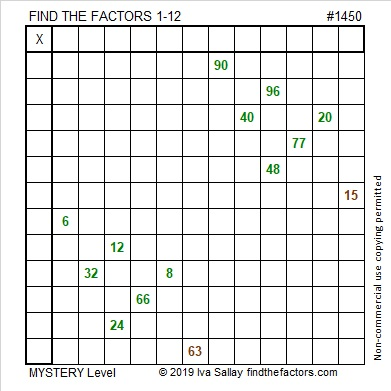Every puzzle has a puzzle number to distinguish it from the others. Here are some facts about this puzzle number, 1450:

• 1450 is a composite number.
• Prime factorization: 1450 = 2 × 5 × 5 × 29, which can be written 1450 = 2 × 5² × 29
• 1450 has at least one exponent greater than 1 in its prime factorization so √1450 can be simplified. Taking the factor pair from the factor pair table below with the largest square number factor, we get √1450 = (√25)(√58) = 5√58
• The exponents in the prime factorization are 1, 2, and 1. Adding one to each exponent and multiplying we get (1 + 1)(2 + 1)(1 + 1) = 2 × 3 × 2 = 12. Therefore 1450 has exactly 12 factors.
• The factors of 1450 are outlined with their factor pair partners in the graphic below.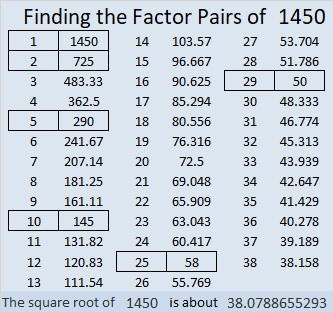In case you are looking for factor trees for 1450, here are two different ones: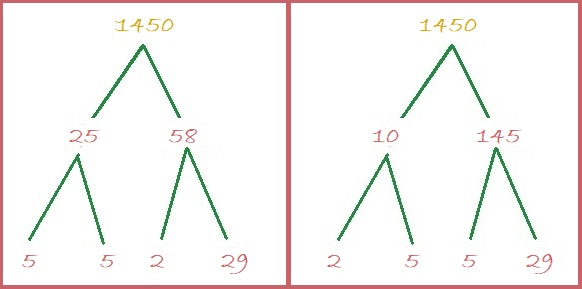1450 is the hypotenuse of SEVEN Pythagorean triples:
170-1440-1450 which is 10 times (17-144-145)
240-1430-1450 which is 10 times (24-143-145)
406-1392-1450 which is (7-24-25) times 58
666-1288-1450 which is 2 times (333-644-725)
728-1254-1450 which is 2 times (364-627-725)
870-1160-1450 which is (3-4-5) times 290
1000-1050-1450 which is (20-21-29) times 50

# 1448 Christmas Factor Tree

Here’s a puzzle that looks a little like a Christmas tree. Some of the clues might give you a little bit of trouble. For example, the common factor of 60 and 30 might be 5, 6, or 10. Likewise, the common factor of 8 and 4 might be 1, 2, or 4.

Which factor should you use? Look at all the other clues and use logic. Logic can help you write each of the numbers 1 to 12 in both the first column and the top row so that the given clues and those numbers behave like a multiplication table. Good luck!I have to number every puzzle. It won’t help you solve the puzzle, but here are some facts about the number 1448:

The number made by its last two digits, 48, is divisible by 4, so 1448 is also divisible by 4. That fact can give us the first couple of branches of 1448’s factor tree: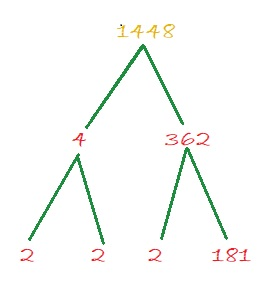• 1448 is a composite number.
• Prime factorization: 1448 = 2 × 2 × 2 × 181, which can be written 1448 = 2³ × 181
• 1448 has at least one exponent greater than 1 in its prime factorization so √1448 can be simplified. Taking the factor pair from the factor pair table below with the largest square number factor, we get √1448 = (√4)(√362) = 2√362
• The exponents in the prime factorization are 3 and 1. Adding one to each exponent and multiplying we get (3 + 1)(1 + 1) = 4 × 2 = 8. Therefore 1448 has exactly 8 factors.
• The factors of 1448 are outlined with their factor pair partners in the graphic below.1448 is also the hypotenuse of a Pythagorean triple:
152-1440-1448 which is 8 times (19-180-181)

# 1308 and Level 6

Can you find the factors that will turn this puzzle into a multiplication table? It’s a level 6 so you might find it to be a challenge. Use logic. Don’t guess and check.Print the puzzles or type the solution in this excel file: 10-factors-1302-1310

Here are a few facts about the puzzle number, 1308:

• 1308 is a composite number.
• Prime factorization: 1308 = 2 × 2 × 3 × 109, which can be written 1308 = 2² × 3 × 109
• The exponents in the prime factorization are 2, 1, and 1. Adding one to each and multiplying we get (2 + 1)(1 + 1)(1 + 1) = 3 × 2 × 2 = 12. Therefore 1308 has exactly 12 factors.
• Factors of 1308: 1, 2, 3, 4, 6, 12, 109, 218, 327, 436, 654, 1308
• Factor pairs: 1308 = 1 × 1308, 2 × 654, 3 × 436, 4 × 327, 6 × 218, or 12 × 109
• Taking the factor pair with the largest square number factor, we get √1308 = (√4)(√327) = 2√327 ≈ 36.16628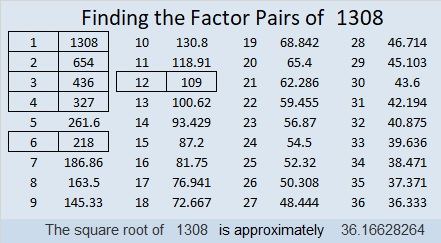1308 is the hypotenuse of a Pythagorean triple:
720-1092-1308 which is 12 times (60-91-109)

As shown in their factor trees below, 1308, 1309, 1310, and 1311 each have three distinct prime numbers in their prime factorizations. They are the smallest set of four consecutive numbers with the same number of prime factors. Thank you OEIS.org for alerting me to that fact.# 996 Christmas Factor Tree

The puzzles this week might look rather plain, but together the seven puzzles make a lovely Christmas tree factoring puzzle. The difficulty level of each of the puzzles is not identified. Some of them are very easy, and some of them are difficult. Some are in-between. How many of them can you solve?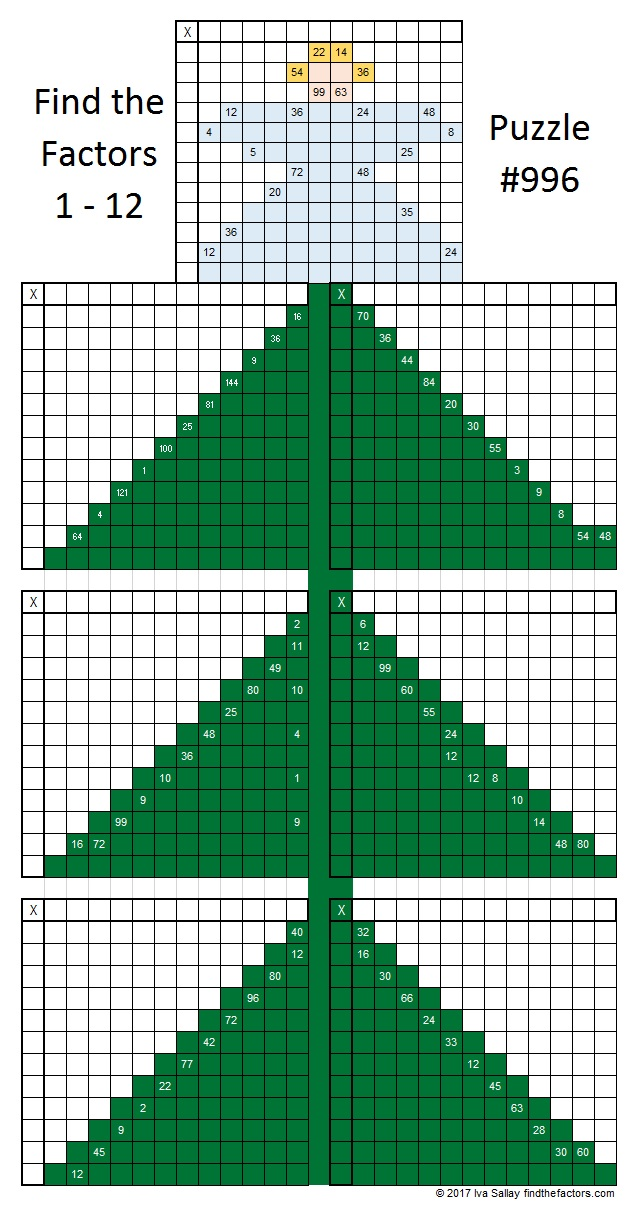Print the puzzles or type the solution in this excel file: 12 factors 993-1001

Here’s a little about the number 996:

Here are a few of its possible factor trees. They look a little like Christmas trees, too.Usually,  I only go up to base 36 when I look for palindromes or repdigits. 966 is NOT a palindrome or repdigit in any of those bases, but can it ever be one? To me, repdigits are more interesting than palindromes because you can find them by factoring.  966 has 6 factors greater than 36: 83, 166, 249, 332, 498, 996. If you subtract 1 from each of those, then 996 will be a repdigit in each of those bases.
In BASE 82, it’s CC (C is 12 base 10)
In BASE 165, it’s 66
In BASE 248, it’s 44
In BASE 331, it’s 33
In BASE 497, it’s 22
In BASE 995, it’s 11
Don’t be surprised when I tell you that 12, 6, 4, 3, 2, and 1 are also factors of 996!

• 996 is a composite number.
• Prime factorization: 996 = 2 × 2 × 3 × 83, which can be written 996 = 2² × 3 × 83
• The exponents in the prime factorization are 2, 1, and 1. Adding one to each and multiplying we get (2 + 1)(1 + 1)(1 + 1) = 3 × 2 × 2 = 12. Therefore 996 has exactly 12 factors.
• Factors of 996: 1, 2, 3, 4, 6, 12, 83, 166, 249, 332, 498, 996
• Factor pairs: 996 = 1 × 996, 2 × 498, 3 × 332, 4 × 249, 6 × 166, or 12 × 83,
• Taking the factor pair with the largest square number factor, we get √996 = (√4)(√249) = 2√249 ≈ 31.55947# 992 Christmas Factor Tree

Artificial Christmas trees have to be assembled. Sometimes the assembly is easy, and sometimes it is frustrating.

This Christmas tree puzzle can be solved using LOGIC and an ordinary multiplication table, but there’s a good chance it will frustrate you. Go ahead and try to solve it!Print the puzzles or type the solution in this excel file: 10-factors-986-992

The number 992 also can make a nice looking, well-balanced factor tree:992 is the product of two consecutive numbers: 31 × 32 = 992.
Because of that fact, 992 is the sum of the first 31 EVEN numbers:
2 + 4 + 6 + 8 + 10 + . . . + 54 + 56 + 58 + 60 + 62 = 992

992 is palindrome 212 in BASE 22 because 2(22²) + 1(22) + 2(1) = 922. That was a lot of 2’s and 1’s in that fun fact!

• 992 is a composite number.
• Prime factorization: 992 = 2 × 2 × 2 × 2 × 2 × 31, which can be written 992 = 2⁵ × 31
• The exponents in the prime factorization are 5 and 1. Adding one to each and multiplying we get (5 + 1)(1 + 1) = 6 × 2 = 12. Therefore 992 has exactly 12 factors.
• Factors of 992: 1, 2, 4, 8, 16, 31, 32, 62, 124, 248, 496, 992
• Factor pairs: 992 = 1 × 992, 2 × 496, 4 × 248, 8 × 124, 16 × 62, or 31 × 32
• Taking the factor pair with the largest square number factor, we get √992 = (√16)(√62) = 4√62 ≈ 31.49603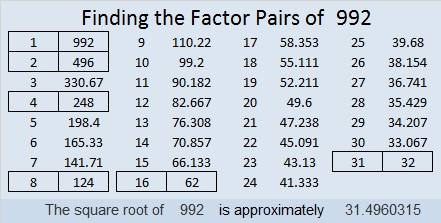# 984 Way Too Big Christmas Factor Tree

Some Christmas trees are so big they are difficult to take home in the car. They might even be too big to set up in the house. This puzzle is the biggest one I have ever made. It looks like a very big Christmas tree waiting to be set up. Is it too big to bring fun this Christmastime?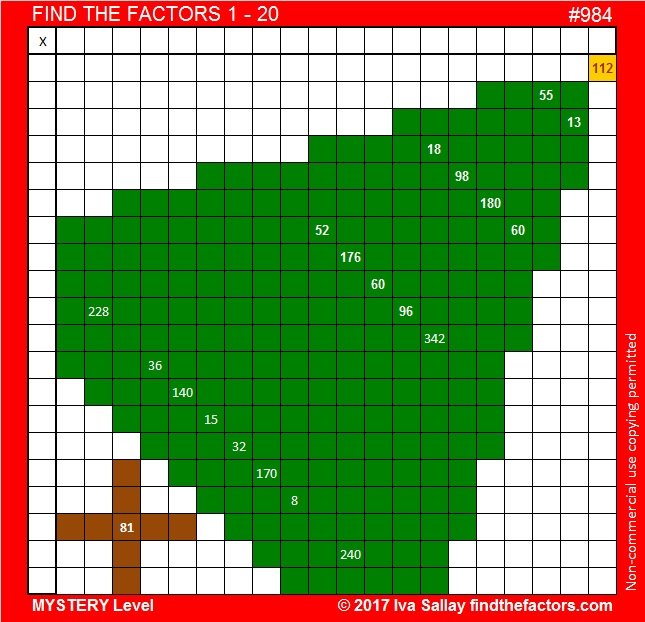The table below may be helpful in solving the puzzle. There are 400 places to write products in a 20 × 20 multiplication table, but not all the numbers from 1 to 400 appear in such a table. Some numbers don’t appear at all while other numbers appear more than one times. The chart below is color-coded to show how many times a product appears in the 20 × 20 multiplication table. Clues in the puzzle that appear only once (yellow) or twice (green) in the multiplication table won’t cause much trouble when solving the puzzle. Any other clues might stump you. Notice that the number 60 appears twice in the puzzle but eight times (black) in the 20 × 20 multiplication table!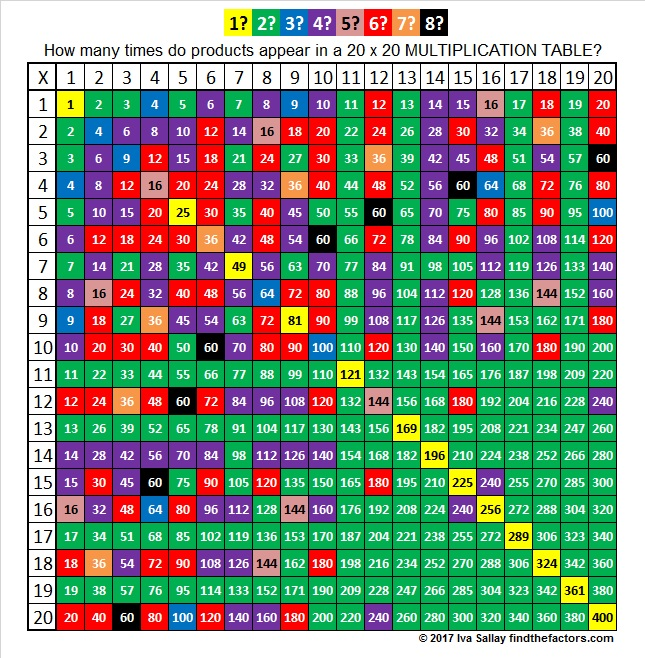I’d like to share some information about the number 984.

It is the hypotenuse of a Pythagorean triple:
216-960-984 which is 24 times (9-40-41)

OEIS.org informs us that 8 + 88 + 888 = 984.

984 is 1313 in BASE 9 because 1(9³) + 3(9²) + 1(9¹) + 3(9⁰) = 984.

Here are a couple of the many possible factor trees for 984: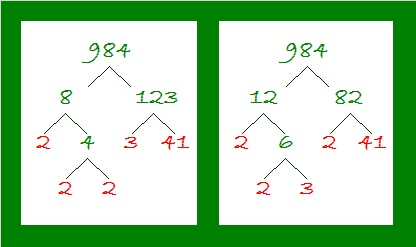• 984 is a composite number.
• Prime factorization: 984 = 2 × 2 × 2 × 3 × 41, which can be written 984 = 2³ × 3 × 41
• The exponents in the prime factorization are 3, 1, and 1. Adding one to each and multiplying we get (3 + 1)(1 + 1)(1 + 1) = 4 × 2 × 2 = 16. Therefore 984 has exactly 16 factors.
• Factors of 984: 1, 2, 3, 4, 6, 8, 12, 24, 41, 82, 123, 164, 246, 328, 492, 984
• Factor pairs: 984 = 1 × 984, 2 × 492, 3 × 328, 4 × 246, 6 × 164, 8 × 123, 12 × 82, or 24 × 41
• Taking the factor pair with the largest square number factor, we get √984 = (√4)(√246) = 2√246 ≈ 31.36877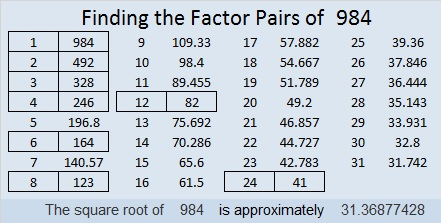# 980 Christmas Factor Trees

This level 4 puzzle has 12 clues in it that are products of factor pairs in which both factors are numbers from 1 to 12. The clues make an evergreen tree, the symbol of everlasting life which is so fitting for Christmas. Can you find the factors for the given clues and put them in the right places?

Now I’ll tell you a little about the number 980:

It has eighteen factors and many possible factor trees. Here are just three of them:28² + 14² = 980, so 980 is the hypotenuse of a Pythagorean triple:
588-784-980 which is (3-4-5) times 196, but can also be calculated from
28² – 14², 2(28)(14), 28² + 14²

I like the way 980 looks in some other bases:
It is 5A5 in BASE 13 (A is 10 base 10) because 5(13) + 10(13) + 5(1) = 980,
500 in BASE 14 because 5(14²) = 980,
SS in BASE 34 (S is 28 base 10) because 28(34) + 28(1) = 28(35) = 980
S0 in BASE 35 because 28(35) = 980

• 980 is a composite number.
• Prime factorization: 980 = 2 × 2 × 5 × 7 × 7, which can be written 980 = 2² × 5 × 7²
• The exponents in the prime factorization are 2, 1 and 2. Adding one to each exponent and multiplying we get (2 + 1)(1 + 1)(2 + 1) = 3 × 2 × 3 = 18. Therefore 980 has exactly 18 factors.
• Factors of 980: 1, 2, 4, 5, 7, 10, 14, 20, 28, 35, 49, 70, 98, 140, 196, 245, 490, 980
• Factor pairs: 980 = 1 × 980, 2 × 490, 4 × 245, 5 × 196, 7 × 140, 10 × 98, 14 × 70, 20 × 49 or 28 × 35
• Taking the factor pair with the largest square number factor, we get √980 = (√196)(√5) = 14√5 ≈ 31.30495.# 702 A Couple of Christmas Factor Trees

Since the sum of its digits equals nine, 702 is divisible by nine.

• 702 is a composite number.
• Prime factorization: 702 = 2 x 3 x 3 x 3 x 13, which can be written 702 = 2 x (3^3) x 13
• The exponents in the prime factorization are 1, 3, and 1. Adding one to each and multiplying we get (1 + 1)(3 + 1)(1 + 1) = 2 x 4 x 2 = 16. Therefore 702 has exactly 16 factors.
• Factors of 702: 1, 2, 3, 6, 9, 13, 18, 26, 27, 39, 54, 78, 117, 234, 351, 702
• Factor pairs: 702 = 1 x 702, 2 x 351, 3 x 234, 6 x 117, 9 x 78, 13 x 54, 18 x 39, or 26 x 27
• Taking the factor pair with the largest square number factor, we get √702 = (√9)(√78) = 3√78 ≈ 26.49528.702 is the product of consecutive integers: 26 x 27 = 702. Numbers that can be expressed as such products are known as Pronic numbers.

It seems only natural to make factor trees based on those two multiplication facts:Today’s Find the Factors puzzle also looks like a couple of small Christmas trees.Print the puzzles or type the solution on this excel file: 12 Factors 2015-11-30

———————————————————————————

Here are more facts about the number 702:

It is the sum of consecutive prime numbers 349 and 353.

It is also the sum of the seventeen prime numbers from 7 to 73.

And because 13 is one of its factors, 702 is the hypotenuse of Pythagorean triple 270-648-702. Notice that the short leg is a permutation of 702.

———————————————————————————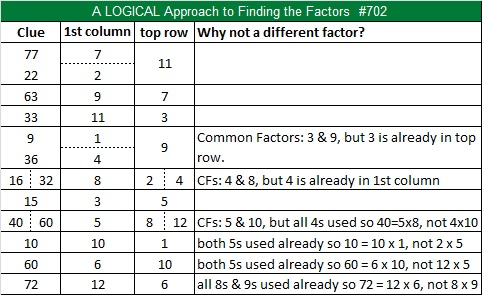# 14 Oh Christmas Tree

14 is a composite number. 14 = 1 x 14 or 2 x 7. Factors of 14: 1, 2, 7, 14. Prime factorization: 14 = 2 x 7.When 14 is a clue in the FIND THE FACTORS  1 – 10 or 1 – 12 puzzles, use 2 and 7 as the factors.

O Christmas Tree, O Christmas Tree,